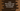# Java program to find square root and cubic root of a number## Write a Java program to find square root and cubic root of a number:

Java has inbuilt methods to find square root and cube root of a number . Both of these methods are already included in the ‘Math’ module.

Following are these methods :

### static double sqrt(double a):

This is a static method and we can find out the square root of a number using it. You can call it directly like ‘Math.sqrt(number)’ as it is a static method of ‘Math’ class. The return value is also ‘double’. If the argument is less than zero than the result will be ‘NaN’. If the argument is ‘-0’ or ‘0’, output will be ‘0.0’.

### public static double cbrt(double a):

‘cbrt’ is a static method and we can find out the cubic root of a number using this method. Similar to ‘sqrt’, this method is also a static method . Argument should be double and the return value is also double. If the argument is negative , then the output is also negative . e.g. cube root of -27 is -3.0 .

Let’s try to understand both of these methods using an example :

``````/*
*
* you may not use this file except in compliance with the License.
* You may obtain a copy of the License at
*
*
* Unless required by applicable law or agreed to in writing, software
* WITHOUT WARRANTIES OR CONDITIONS OF ANY KIND, either express or implied.
* See the License for the specific language governing permissions and
*/

import java.util.Scanner;

/**
* Example class
*/
public class ExampleClass {

//utility method to print a string
static void print(String value) {
System.out.println(value);
}

public static void main(String[] args) {
Scanner scanner = new Scanner(System.in);

int userInput;
print("Enter a number to find the cube-root : ");

userInput = scanner.nextInt();

print("Cube root is : "+Math.cbrt(userInput));

print("Enter a number to find the square-root : ");
userInput = scanner.nextInt();

print("Square root is : "+Math.sqrt(userInput));

}

}``````

### Sample Example output :

``````Enter a number to find the cube-root :
27
Cube root is : 3.0
Enter a number to find the square-root :
4
Square root is : 2.0

Enter a number to find the cube-root :
-8
Cube root is : -2.0
Enter a number to find the square-root :
-144
Square root is : NaN

Enter a number to find the cube-root :
-0
Cube root is : 0.0
Enter a number to find the square-root :
-0
Square root is : 0.0``````